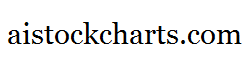Members Area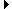Sign Up | Log In
 Free Level 2 QuotesStock AnalysisCorrelation Trading PlatformCorrelationsMembership Benefits

BB (Bollinger Bands)
 Lookup a different indicator.

 Indicator Usage Description Example bb bb[x,y,z,n] OPERATOR (INDICATOR or NUMBER) , where x is a positive integer >= 2 representing the x-day sma period, y is a positive integer from 2 to 10 representing the distance from the sma, z is either 'u' or 'l' representing 'upper' and 'lower' Bollinger Bands and n is optional and represents the number of days ago the result is computed (n=0 is the default value and represents the current end of day, n=1 is the previous end of day, etc.) Returns the x,y,z Bollinger Band value from n days ago. (close > bb[20,2,u])

Introduction:

Bollinger Bands are lines that are drawn equidistant from a simple moving average. They attempt to define relative high and low stock prices by considering the variation of the stock price through the use of a standard deviation multiplier. Bollinger Bands were introduced by John Bollinger in the early 1980's and are widely used in stock charting packages.

Example:

The following chart of Intel (INTC) highlights days where the closing price surpasses the 20,2 upper Bollinger Band.

 Chart of Intel (INTC) highlighting days where the closing price surpasses the 20,2 upper Bollinger Band.

INDICATORS IN OUR DATABASE THAT INCLUDE THE BB INDICATOR...

Click here to view all indicators that include the indicator 'bb' (sorted by AISCORE)

 (((bb[20,2,u] - bb[20,2,l]) / sma) < 0.1)AISCORE: 2519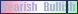Indicator ID: 060827190849(((bb[20,2,u] - bb[20,2,l]) / sma) < 0.05)AISCORE: 2057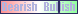Indicator ID: 060827193016(((bb[20,2,u] - bb[20,2,l]) / sma) < 0.07)AISCORE: 1257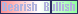Indicator ID: 060827194642(((bb[20,2,u] - bb[20,2,l]) / sma) < 0.06)AISCORE: 2360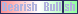Indicator ID: 060827195233(((bb[20,2,u] - bb[20,2,l]) / sma) < 0.08)AISCORE: 1725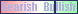Indicator ID: 060827195523 More ->